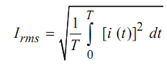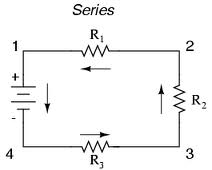## RMS and Average Value Assignment Help

Assignment Help: >> Introduction to Alternating Quantity - RMS and Average Value

RMS and Average Value:

These two values represent the direct value (steady values) of any alternating quantity and described as:

RMS Value (Root Mean Square Value)

The root mean square current is given by that equivalent steady (dc) current which shall generated the same amount of heat in a given circuit as could be generated by the alternating current when flowing through the same circuit for the similar time period.

Consider the circuit illustrated in Figure. First we connect the Direct Source (DC) to the resistor for the specific time t and find the heat i2 Rt joules. Then for the same time t, we apply the alternating current from AC source v and again compute the heat transferred to the resistor. Now, the RMS value of current i is given by,T = Time period of current wave.

RMS value is also known as the effective or virtual value.Average Value Average Value of Sinusoidal Wave Form Factor RMS Value of Complex Wave RMS Value of Sinusoidal Wave#### Assured A++ Grade

Get guaranteed satisfaction & time on delivery in every assignment order you paid with us! We ensure premium quality solution document along with free turntin report!

All rights reserved! Copyrights ©2019-2020 ExpertsMind IT Educational Pvt Ltd